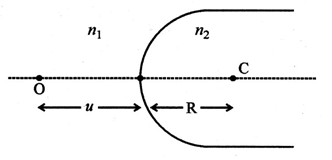(a) A point object 'O' is kept in a medium of refractive index            (5 marks)

n1 in front of a convex spherical surface of radius of curvature

R which separates the second medium of refractive index n2

from the first one, as shown in the figure.

Draw the ray diagram showing the image formation and deduce

the relationship between the object distance and the image distance

in terms of n1, n2 and R.(b) When the image formed above acts as a virtual object for concave

spherical, surface separating the medium n2 from n1 (n2 > n1), draw

this ray diagram and write the similar (similar to (a)) relation. Hence

obtain the expression for the lens maker's formula.